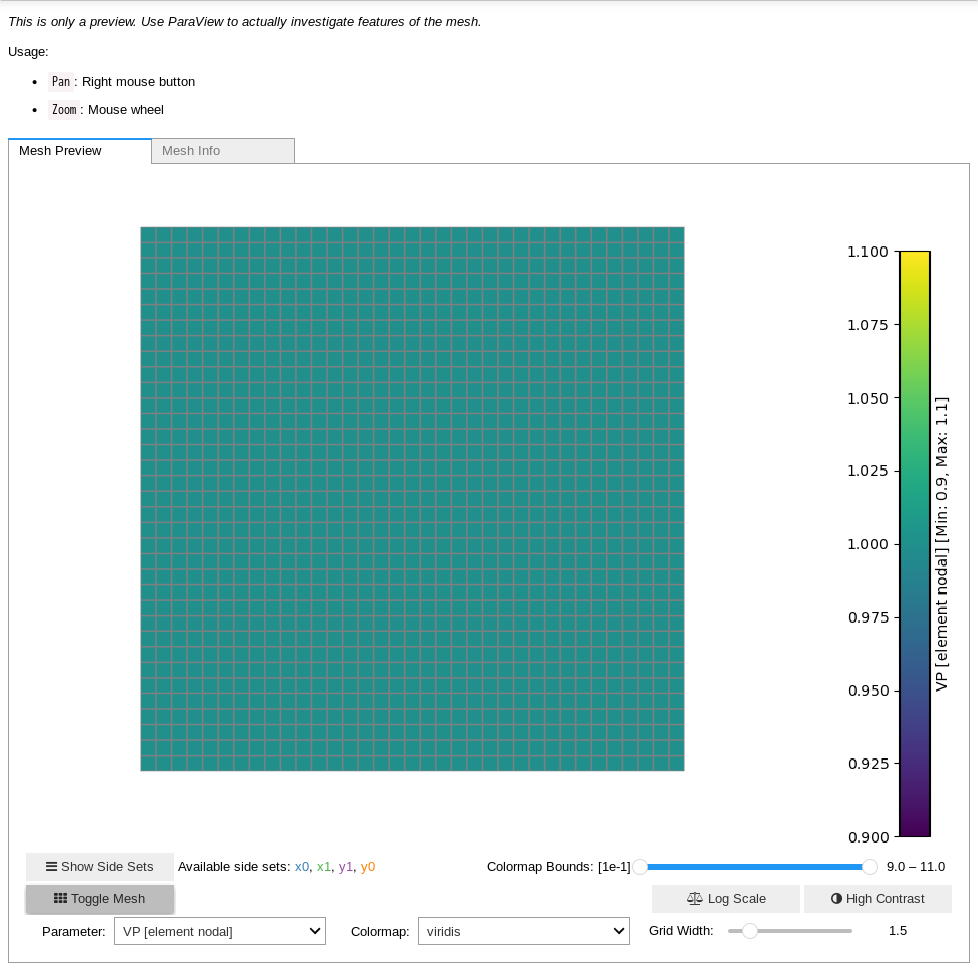Version:This tutorial is presented as Python code running inside a Jupyter Notebook, the recommended way to use Salvus. To run it yourself you can copy/type each individual cell or directly download the full notebook, including all required files.# Working with Salvus' wavefield output files

Salvus contains a powerful set of tools that enable users to output simulated dynamic quantities, such as velocity, displacement, or strain, within volumes and along surfaces. These outputs are, in general, saved on unstructured meshes, making them somewhat awkward to work with using standard tools such as numpy or xarray. To remedy this, Salvus contains a lightweight WavefieldOutput class that enables the simple extraction of stored quantities to regular grids. Let's dive in and take a look.
Copy
import os

import numpy as np
import salvus.namespace as sn
from salvus.mesh import layered_meshing as lm
from salvus.toolbox.helpers import wavefield_output

SALVUS_FLOW_SITE_NAME = os.environ.get("SITE_NAME", "local")

## Setting up a simple simulation

We'll demonstrate the basic concepts with a 2-D isotropic elastic mesh created using Salvus' layered_meshing API. The use of this API, however, is not required to use the functionality presented herein, and any volumetric simulation output from a Salvus simulation will suffice.
material = lm.material.elastic.Velocity.from_params(
rho=1.0, vp=1.0, vs=np.sqrt(1 / 3)
)

mesh_2d = lm.mesh_from_domain(
sn.domain.dim2.BoxDomain(x0=0, x1=1, y0=0, y1=1),
material,
sn.MeshResolution(reference_frequency=10.0, elements_per_wavelength=2.0),
)

mesh_2d<salvus.mesh.unstructured_mesh.UnstructuredMesh at 0x7fd60af85e10>
We'll then create a source at the top of the domain ...
stf = sn.simple_config.stf.Ricker(center_frequency=2.5)
src_2d = sn.simple_config.source.cartesian.VectorPoint2D(
x=0.5, y=1.0, fx=0.0, fy=-1.0, source_time_function=stf
)
... and set up a simple simulation.
w_2d = sn.simple_config.simulation.Waveform(
mesh=mesh_2d, sources=src_2d, end_time_in_seconds=2.0
)

w_2d